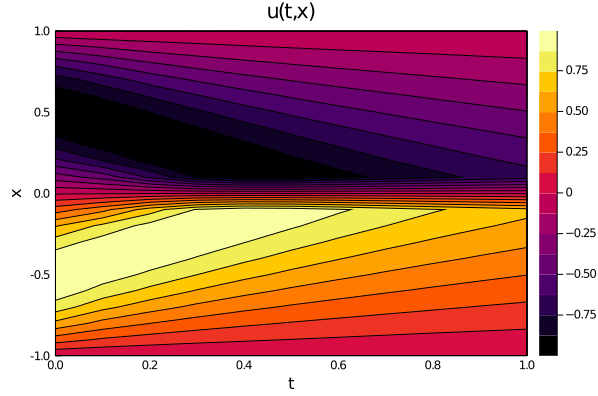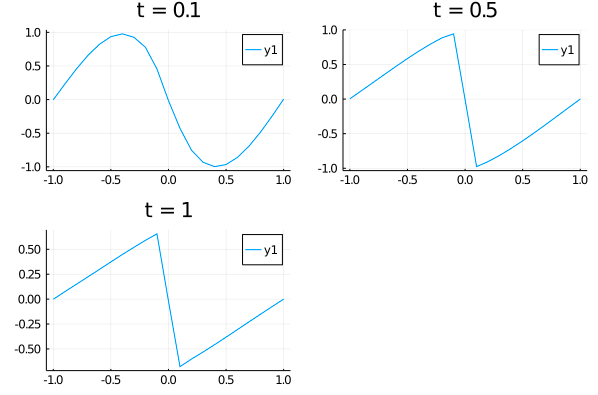# Investigating symbolic_discretize with the 1-D Burgers' Equation

Let's consider the Burgers' equation:

$$$\begin{gather*} ∂_t u + u ∂_x u - (0.01 / \pi) ∂_x^2 u = 0 \, , \quad x \in [-1, 1], t \in [0, 1] \, , \\ u(0, x) = - \sin(\pi x) \, , \\ u(t, -1) = u(t, 1) = 0 \, , \end{gather*}$$$

with Physics-Informed Neural Networks. Here is an example of using the low-level API:

using NeuralPDE, Lux, ModelingToolkit, Optimization, OptimizationOptimJL
import ModelingToolkit: Interval, infimum, supremum

@parameters t, x
@variables u(..)
Dt = Differential(t)
Dx = Differential(x)
Dxx = Differential(x)^2

#2D PDE
eq  = Dt(u(t,x)) + u(t,x)*Dx(u(t,x)) - (0.01/pi)*Dxx(u(t,x)) ~ 0

# Initial and boundary conditions
bcs = [u(0,x) ~ -sin(pi*x),
u(t,-1) ~ 0.,
u(t,1) ~ 0.,
u(t,-1) ~ u(t,1)]

# Space and time domains
domains = [t ∈ Interval(0.0,1.0),
x ∈ Interval(-1.0,1.0)]

# Discretization
dx = 0.05

# Neural network
chain = Lux.Chain(Dense(2,16,Lux.σ),Dense(16,16,Lux.σ),Dense(16,1))
strategy = NeuralPDE.GridTraining(dx)

indvars = [t,x]
depvars = [u(t,x)]
@named pde_system = PDESystem(eq,bcs,domains,indvars,depvars)

discretization = PhysicsInformedNN(chain, strategy)
sym_prob = symbolic_discretize(pde_system,discretization)

phi = sym_prob.phi

pde_loss_functions = sym_prob.loss_functions.pde_loss_functions
bc_loss_functions = sym_prob.loss_functions.bc_loss_functions

callback = function (p, l)
println("loss: ", l)
println("pde_losses: ", map(l_ -> l_(p), pde_loss_functions))
println("bcs_losses: ", map(l_ -> l_(p), bc_loss_functions))
return false
end

loss_functions =  [pde_loss_functions;bc_loss_functions]

function loss_function(θ,p)
sum(map(l->l(θ) ,loss_functions))
end

f_ = OptimizationFunction(loss_function, Optimization.AutoZygote())
prob = Optimization.OptimizationProblem(f_, sym_prob.flat_init_params)

res = Optimization.solve(prob,OptimizationOptimJL.BFGS(); callback = callback, maxiters=2000)

And some analysis:

using Plots

ts,xs = [infimum(d.domain):dx:supremum(d.domain) for d in domains]
u_predict_contourf = reshape([first(phi([t,x],res.u)) for t in ts for x in xs] ,length(xs),length(ts))
plot(ts, xs, u_predict_contourf, linetype=:contourf,title = "predict")

u_predict = [[first(phi([t,x],res.u)) for x in xs] for t in ts ]
p1= plot(xs, u_predict,title = "t = 0.1");
p2= plot(xs, u_predict,title = "t = 0.5");
p3= plot(xs, u_predict[end],title = "t = 1");
plot(p1,p2,p3)